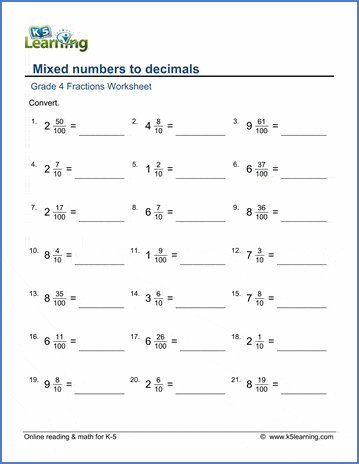# Fractions to/from Decimals for Grade 4

## Converting fractions to and from decimals worksheets

These worksheets provide introductory exercises for converting fractions to and from decimals.  All fractions have a denominator of 10 or 100 for easy translation into decimals.  Mixed numbers are also used.

## Decimals to fractions

Decimals to fractions (tenths/hundredths) 0.56 =
Decimals to mixed numbers (tenths/hundredths) 5.12 =

## Fractions to decimals

Fractions to decimals 73/100 =
Mixed numbers to decimals 7 7/10 =Sample Grade 4 converting fractions to decimals worksheet

## More decimals worksheets

Find all of our decimals worksheets, from converting fractions to decimals to long division of multi-digit decimal numbers.

## More fractions worksheets

Explore all of our fractions worksheets, from dividing shapes into "equal parts" to multiplying and dividing improper fractions and mixed numbers.

What is K5?

K5 Learning offers free worksheets, flashcards and inexpensive workbooks for kids in kindergarten to grade 5. Become a member to access additional content and skip ads.